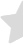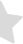# How to Measure the Thickness of Paper

by Chris & Marry

From packaging to the different uses of paper, paper thickness can be a crucial thing to familiarize yourself with. Thickness determines the durability, strength, and usage of the paper overall. But the question that arises is: how can we measure the thickness of paper? A single sheet of paper is generally relatively thin, so how is it possible to measure its thickness? Surely, a ruler would not be able to do the job? The answer to that might come as a baffling one: yes! It is quite possible to measure the thickness of paper with a ruler, and this is how:

## Measuring the Thickness of Paper with a Ruler

While measuring the length and breadth of a sheet of paper using a ruler is no hassle at all, finding out the thickness can be tricky. But what we can do to figure out the thickness of a single sheet of paper is simply stacking 100 sheets of paper and making a bundle out of them. Using a ruler, the stack of 100 sheets of paper can be measured relatively easily. So, now that you have the measurement for 100 sheets of paper, by simple arithmetic, the thickness of a single sheet of paper can be calculated. You can do this by dividing the height of the paper bundles by the number of sheets used to make the bundle. For example, if 100 sheets of paper are used to create a bundle and using a ruler, the height of the bundle was found to be 10mm, then the thickness of a single sheet of paper is going to be 0.1mm.

While this method is an easy one, it is not exactly recommended. The likelihood of inaccuracy is mostly large with this one, but it's perhaps the easiest method out there.

## Measuring the Thickness of Paper using a Caliper

A caliper can measure the thickness of paper in a relatively more accurate way compared to using just a ruler. Firstly, let's familiarise ourselves with what a caliper is:

A caliper is a device that is used to measure external or internal dimensions. Using this, we should be able to measure the thickness of the paper accurately. A caliper contains two jaws that can hold the paper, and a reading can be calculated.

Initially, calibrate the caliper, setting it to either mm or inches. Reset it and bring about a standard measurement by rotating the wheel on the caliper piece and set it to 0, assuming the caliper is an electronic one. Measure anything random like a stone or spoon and reset it back to 0. Doing this will ensure the caliper gets accurate results.

Secondly, get around 100 pieces of paper and stack them on top of each other. Using the caliper, make adjustments and match the thickness of the ream of the stack of papers being measured. Rotating the jaws will help fit the ream inside. Secure the stack of papers, but not too tight to not tear or crush down. This can increase measurement inaccuracy. Assuming the caliper is electronic, the measurement will show up on the display panel. This will give you the thickness measurement of the whole stack. From this, you can calculate for a single sheet of paper like we have done when measuring with a ruler.

An example: Say the measurement on the caliper shows up as 1mm or 1 inch. Divide this 1mm or inch with the number of papers stacked. So if the number of papers stacked is 100, the thickness of a single sheet of paper will be 1/100 = 0.01mm or inch.

## Measuring the Thickness of Paper using a Micrometer

Alternatively to the two methods mentioned above, a micrometer can be used to measure the thickness of paper as well. It is a lot more accurate than using a caliper and it also requires more precision when using it.

So, what is a micrometer? It's a caliper of some sort but for micro-measurements. This means the measurements of the thickness of paper are bound to be a lot more accurate than the measurements you may get with a ruler and a caliper.

To use a micrometer, move the dial on the micrometer to adjust its jaws. This will move the shafts of the micrometer up and down accordingly. Measure anything to test the micrometer and see whether everything can be read easily from the micrometer. Once the micrometer has been successfully calibrated, you can proceed to measure the thickness of the paper.

Stack around 100 sheets of paper, set the micrometer up at the ideal thickness of the paper, and observe how the shaft moves upon how much the jaw opens. Adjust the jaws and get the marks on the shaft gauge. The shaft gauge is a set of lines going from 0 to 25. The shaft gauge measure should go perfectly with the lines on the tube shaft.

Get the first measurement by making adjustments to the jaws of the micrometer to the stack of papers. Observe which lines on the gauge go hand in hand with the opposite lines of the shaft tube to get the second measurement. The lines will give you the information on the paper stack thickness. The two measurements will enable you to calculate the thickness of the paper.

Add the two numbers. The resulting number will give you the total thickness of the paper stack. Now, divide this number by the number of sheets used. So, if we use 100 sheets of paper in our stack and the total thickness we get is 0.106 inches, then the thickness of a single sheet of paper will be 0.106/100 = 1.06 x 10^-3 inches per sheet.

## Expert Tips for Measuring the Thickness of Paper

There are a number of reasons why measuring the thickness of paper is important. Paper thickness can affect everything from the printing process to the durability of the finished product.

One of the most important reasons to measure paper thickness is to ensure that it is compatible with the printing process. The thickness of paper can impact a number of factors in the printing process, including the amount of ink that is required and the clarity of the final print. If paper is too thick, it can cause problems with ink absorption and result in a muddy or unclear print. On the other hand, if paper is too thin, it may tear during the printing process or end up with an uneven finish.

Paper thickness can also affect how durable a printed product is. If a printed piece is too thin, it is more likely to tear or be damaged during use. If a piece is too thick, it may be difficult to fold or crease without damaging the paper. Measuring paper thickness can help ensure that a printed product will be able to withstand the rigors of use.

In some cases, measuring paper thickness is also important for aesthetic reasons. Some papers have different textures or patterns that can be achieved by varying the thickness of the paper. By measuring the thickness of the paper, printers can ensure that they are using the right type of paper to create the desired effect.

## How to Calculate the Weight per Unit Area of Paper ?

Weight per unit area of paper is a key performance parameter in the printing and packaging industry. It is a function of both the basis weight of the paper and the caliper or thickness of the sheet. Basis weight is defined as the weight in pounds of a ream (500 sheets) of paper cut to the basic size for that grade. "Caliper" is a term used to describe the thickness of paper and is measured in thousandths of an inch. The term "basis weight" actually refers to two separate but related measures. One is the actual weight in pounds of 500 sheets of paper cut to its basic size; this is also called the "parent size." The other basis weight measure, commonly referred to as the "basis size," is the ream weight divided by the basic size.

The most common way of expressing basis weight is pounds per thousand square feet (MSF). For example, a basis weight of 40 MSF means that a ream of 500 sheets of paper weighs 20 pounds and has an area of 1,000 square feet. The term "caliper" is a measure of paper thickness and is usually expressed in thousandths of an inch (mils). For example, a sheet with a caliper of 3 mils has a thickness of 3/1000th of an inch or 0.003 inches.

Basis weight and caliper are related but distinct measures. To calculate the weight per unit area (WPUA) of paper, you need to know both the basis weight and the caliper. The formula for calculating WPUA is:

WPUA = (Basis Weight / Caliper) x 12

For example, if you have a sheet of paper with a basis weight of 40 MSF and a caliper of 3 mils, the calculation would be:

WPUA = (40 / 3) x 12 = 160 lbs/3000 ft2 or 53.3 gsm/m2

It's important to note that the formula for calculating WPUA only works for sheets of paper with uniform basis weights and calipers. If the basis weight or caliper varies across the sheet, you'll need to use a different method to calculate the WPUA.

In general, the higher the basis weight and the caliper, the higher the WPUA. This is because heavier and thicker sheets of paper have more mass per unit area. However, it's also important to consider how the basis weight and caliper affect other properties of paper such as stiffness, opacity, and printability. For example, a sheet with a high basis weight but low caliper may be very stiff and difficult to print on, while a sheet with a low basis weight but high caliper may be very flimsy and prone to tearing.

## Conclusion

While all three methods can measure the thickness of paper, the most accurate one would be using the micrometre method. If you want an easy method, the ruler method is a viable option. So, while measurements are easier to make with just a ruler, using a micrometre would be a better alternative option to avoid any risks of making inaccuracy.

Related posts: